# NCERT Solutions for Class 12 Maths Chapter 9: Differential Equations

In this page we have NCERT Solutions for Class 12 Maths Chapter 9: Differential Equations for EXERCISE 9.1 . Hope you like them and do not forget to like , social share and comment at the end of the page.
Determine order and degree (if defined) of differential equation
Question 1)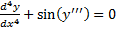Question 2)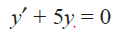Question 3)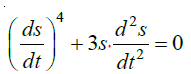Question 4)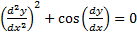Question 5)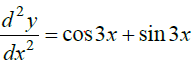Question 6)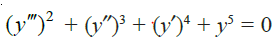Question 7)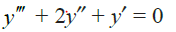Question 8)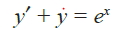Question 9)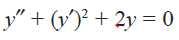Question 10)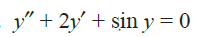Solutions

 S.no Order Degree 1 The highest order derivative present in the differential equation is y””. So, its order is four The given differential equation is not a polynomial equation in its derivatives. Hence, its degree is not defined 2 The highest order derivative present in the differential equation is  dy/dx . So, its order is one. It is a polynomial equation in dy/dx . The highest power raised to is 1. Hence, its degree is one 3 The highest order derivative present in the given differential equation is d2s/dt2. So, its order is two It is a polynomial equation in d2s/dt2 and ds/dt . The power raised to d2s/dt2   is 1. Hence, its degree is one 4 The highest order derivative present in the given differential equation is d2y/dx2  .  So, its order is 2 The given differential equation is not a polynomial equation in its derivatives. Hence, its degree is not defined 5 The highest order derivative present in the differential equation is d2y/dx2   . So, its order is two It is a polynomial equation in d2y/dx2    and the power raised to d2y/dx2    is 1. Hence, its degree is one 6 The highest order derivative present in the differential equation is  y’’’. So, its order is three The given differential equation is a polynomial equation in  y’’’ , y’’ and y’ The highest power raised to is 2. Hence, its degree is 2 7 The highest order derivative present in the differential equation is  y’’’. So, its order is three The given differential equation is a polynomial equation in  y’’’ , y’’ and y’ The highest power raised to is 1. Hence, its degree is 1 8 The highest order derivative present in the differential equation is  y’ . So, its order is one. The given differential equation is a polynomial equation in y’  and  the highest power raised to is one. Hence, its degree is one. 9 The highest order derivative present in the differential equation is y’’ . So, its order is two. The given differential equation is a polynomial equation in y’’ and y’  and the highest power raised to is one. Hence, its degree is one. 10 The highest order derivative present in the differential equation is y’’ . So , its order is two. Degree is one

Question 11)
The degree of the differential equation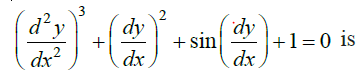(A) 3
(B) 2
(C) 1
(D) not defined
Solution
The given differential equation is not a polynomial equation in its derivatives. Therefore,
its degree is not defined.
Hence, the correct answer is D.

Question 12)
The order of the differential equation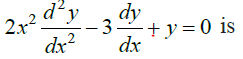(A) 2
(B) 1
(C) 0
(D) not defined
Solution
The highest order derivative present in the given differential equation is d2y/dx2  . Therefore, its order is two.
So, the correct answer is A.

## Related Topics

Go back to Class 12 Main Page using below links